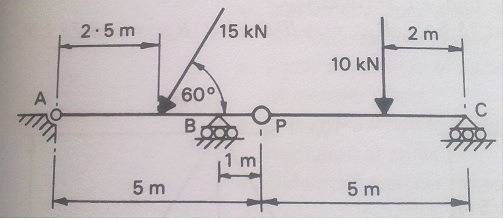# Pinned supported frames help.

## Homework Statement

The beam ABC in attachment has a pinned support at A and Roller supports at B and C. P is an internal pin. I have to determine the reactions at A, B and C and at the pin P when the beam is loaded as shown in the attachment## Homework Equations

$$\Sigma$$V=O $$\Sigma$$H=0

## The Attempt at a Solution

I have split the problem in half and started with the right hand side. I have worked out the reaction at the pin as +4.00 kN and the reaction at C as +6.00 kN. I have done this by taking the moments of P as (10x3)-VC*5=0. I then worked out the sum of the vertical which = 0 to get the reaction at the pin that was VP-VC-10= 0 meaning that VP =+4.00 kN. I have worked out the horizontals on the left by doing the sum of the horizontals = 0, $$\Sigma$$H=0
=HA-15cos60=0 HA= +7.5kN. What I would like to know is how to find out the vertical reaction at A and B Thanks

## Answers and Replies

nvn
Science Advisor
Homework Helper
andycampbell1: Nice work, so far. Hint 1: For the left-hand side, take moments about point A or B.

I have tried taking the moments of A. The book I am working from gives me the answers for VA as +3.87kN and VB as +13.12kN. For taking moments about A I tried this

$$\Sigma$$MA=0
=2.5x15sin(60)-VBx4=0.
I have also tried adding VBx4 and adding the value of the pin and dividing by the length of 5 but I cannot come up with what the book states as the answer. Would I be aswell to keep this as 2 separate structures for taking the moments of A or using the whole structure?

nvn
Science Advisor
Homework Helper
Keeping this as two separate structures for taking moments is good, if you wish. Either way you prefer is fine. Your MA summation, above, omitted VP. You cannot omit forces on your structure (or on your sectioned-off portion of a structure). Also, see the last paragraph of post https://www.physicsforums.com/showpost.php?p=2946515".

Last edited by a moderator:
Hi finally managed to get it, this is what I done

$$\Sigma$$MA=0
=(2.5x15sin60)+(VPx5)-VBx4)=0
=(32.48)+(20)-(VBx4)=0
VB=+13.12 kN

$$\Sigma$$V=0
=VA+VB-15sin60-VP=0
= 0 + 13.12 - 12.99 - 4 = 0
VA = +3.87 kN

Thanks for the links they have helped me.